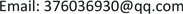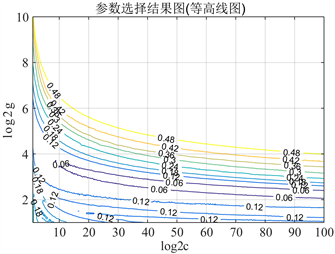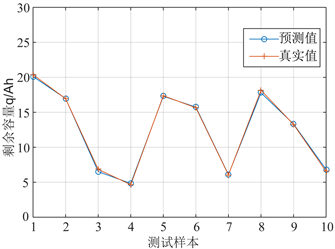﻿ LIBSVM在铅酸蓄电池寿命预测中的应用研究 Application of LIBSVM in Life Prediction of Lead-Acid Battery

Smart Grid
Vol.07 No.05(2017), Article ID:22407,8 pages
10.12677/SG.2017.75045

Application of LIBSVM in Life Prediction of Lead-Acid Battery

Chuankai Yang1,2, Jicheng Zhou3, Wei Liu4, Xu Li4, Liangshu Li5, Feng Fu5, Kai Chen6

1State Gird Shaanxi Electric Power Research Institute, Xi’an Shaanxi

2School of Microelectronics, Xidian University, Xi’an Shaanxi

3School of Electrical Engineering, Wuhan University, Wuhan Hubei

4State Gird Shaanxi Electric Power Company, Xi’an Shaanxi

5State Gird Weinan Power Supply Company, Weinan Shaanxi

6Wuhan Nari Limited Liability Company, State Grid Electric Power Research Institute, Wuhan HubeiReceived: Oct. 4th, 2017; accepted: Oct. 17th, 2017; published: Oct. 23rd, 2017ABSTRACT

As the core of the DC power supply system, the performance of the lead-acid battery is the safe and stable operation of the whole substation. With the use of lead-acid battery pack time increases, the battery’s internal resistance will increase, the battery capacity will be reduced, resulting in lead-acid battery life. Therefore, it is of great significance to study the life prediction of lead-acid battery. Based on the basic principle of support vector machine (SVM) and the sample data of lead-acid battery, the optimal parameters of LIBSVM regression machine are selected by cross validation, and the life prediction model of lead-acid battery is established by support vector machine regression model. The experimental results show that the LIBSVM-based lead-acid battery life prediction model has high prediction accuracy, and the method is feasible.

Keywords:LIBSVM, Lead-Acid Battery, Life Prediction, Substation, Support Vector Machine

LIBSVM在铅酸蓄电池寿命预测中的应用研究

1国网陕西省电力公司电力科学研究院，陕西 西安

2西安电子科技大学微电子学院，陕西 西安

3武汉大学电气工程学院，湖北 武汉

4国网陕西省电力公司，陕西 西安

5国网陕西省电力公司渭南供电公司，陕西 渭南

6国网电力科学研究院武汉南瑞有限责任公司，湖北 武汉Copyright © 2017 by authors and Hans Publishers Inc.1. 引言

2. SVM基本原理

SVM是在统计学习的基础上发展起来的一种新的机器学习方法，在统计学习理论的VC理论和结构风险最小化原则的基础上，SVM能够根据有限样本信息，在模型的复杂性和学习能力之间寻求最佳折衷，避免了局部极小值点。同时，SVM避免了人工神经网络常常出现的“过学习”问题，具有良好的推广性能和较好的分类精确性，对于有限训练样本得到的决策规则对独立的测试集仍然能够得到小的误差。SVM最初被用于分类问题，后来在函数逼近和回归预测等方面也取得了良好的效果。

$S=\left\{\left({x}_{i},{y}_{i}\right)|{x}_{i}\in {R}^{n},y\in R,i=1,2,\cdots ,l\right\}$ (1)

$|{y}_{i}-f\left({x}_{i}\right)|\le \epsilon ,i=1,2,\cdots ,l$ (2)

${d}_{i}=\frac{|\left(w,x\right)+b-{y}_{i}|}{\sqrt{1+{‖w‖}^{2}}}$ (3)

$|\left(w,x\right)+b-{y}_{i}|\le \epsilon ,i=1,2,\cdots ,l$ (4)

${d}_{i}=\frac{|\left(w,x\right)+b-{y}_{i}|}{\sqrt{1+{‖w‖}^{2}}}\le \frac{\epsilon }{\sqrt{1+{‖w‖}^{2}}},i=1,2,\cdots ,l$ (5)

$\begin{array}{l}\mathrm{min}\frac{1}{2}{‖w‖}^{2}\\ \text{s}\text{.t}\text{.}\text{\hspace{0.17em}}\text{\hspace{0.17em}}|\left(w,x\right)+b-{y}_{i}|\le \epsilon ,i=1,2,\cdots ,l\end{array}$ (6)

$L\left(w,b,a,{a}^{*}\right)=\frac{1}{2}{‖w‖}^{2}-\underset{i=1}{\overset{l}{\sum }}{a}_{i}\left(\epsilon -{y}_{i}+\left(w,{x}_{i}\right)+b\right)-\underset{i=1}{\overset{l}{\sum }}{a}_{i}^{*}\left(\epsilon +{y}_{i}-\left(w,{x}_{i}\right)-b\right)$ (7)

Lagrange对偶问题为：

$\underset{a,a*}{\mathrm{max}}\underset{w,b}{\mathrm{min}}L\left(w,b,a,{a}^{*}\right)$ (8)

$\frac{\partial L}{\partial w}=0,\text{\hspace{0.17em}}\text{\hspace{0.17em}}\frac{\partial L}{\partial b}=0$ (9)

$w=\underset{i=1}{\overset{l}{\sum }}\left({a}_{i}-{a}_{i}^{*}\right){x}_{i}$ (10)

$\underset{i=1}{\overset{l}{\sum }}\left({a}_{i}-{a}_{i}^{*}\right)=0$ (11)

$\begin{array}{l}\underset{a,a*}{\mathrm{min}}\frac{1}{2}\underset{i=1,j=1}{\overset{l}{\sum }}\left({a}_{i}^{*}-{a}_{i}\right)\left({a}_{j}^{*}-{a}_{j}\right)\phi \left({x}_{i}-{x}_{j}\right)+\epsilon \underset{i=1}{\overset{l}{\sum }}\left({a}_{i}^{*}-{a}_{i}\right)-\underset{i=1}{\overset{l}{\sum }}{y}_{i}\left({a}_{i}^{*}-{a}_{i}\right)\\ \text{s}\text{.t}\text{.}\text{\hspace{0.17em}}\text{\hspace{0.17em}}\underset{i=1}{\overset{l}{\sum }}\left({a}_{i}^{*}-{a}_{i}\right)=0\\ \text{\hspace{0.17em}}\text{\hspace{0.17em}}\text{\hspace{0.17em}}\text{\hspace{0.17em}}\text{\hspace{0.17em}}\text{\hspace{0.17em}}{a}_{i}^{*},{a}_{i}\ge 0,i=1,2,\cdots ,l\end{array}$ (12)

${\xi }_{i},{\xi }_{i}^{*}\ge 0,i=1,2,\cdots ,l$ (13)

$\begin{array}{l}\mathrm{min}\frac{1}{2}{‖w‖}^{2}+\gamma \underset{i=1}{\overset{l}{\sum }}\left({\xi }_{i}+{\xi }_{i}^{*}\right)\\ \text{s}\text{.t}\text{.}\text{\hspace{0.17em}}\text{\hspace{0.17em}}\left(w,\phi \left({x}_{i}\right)\right)+b-{y}_{i}\le {\xi }_{i}^{*}+\epsilon ,i=1,2,\cdots ,l\\ \text{\hspace{0.17em}}\text{\hspace{0.17em}}\text{\hspace{0.17em}}\text{\hspace{0.17em}}\text{\hspace{0.17em}}\text{\hspace{0.17em}}{y}_{i}-\left(w,\phi \left({x}_{i}\right)\right)-b\le {\xi }_{i}^{*}+\epsilon ,i=1,2,\cdots ,l\\ \text{\hspace{0.17em}}\text{\hspace{0.17em}}\text{\hspace{0.17em}}\text{\hspace{0.17em}}\text{\hspace{0.17em}}\text{\hspace{0.17em}}{\xi }_{i},{\xi }_{i}^{*}\ge 0,i=1,2,,\cdots ,l\end{array}$ (14)

$\begin{array}{c}L\left(w,b,a,{a}^{*}\right)=\frac{1}{2}{‖w‖}^{2}+\gamma \underset{i=1}{\overset{l}{\sum }}\left({\xi }_{i}+{\xi }_{i}^{*}\right)-\underset{i=1}{\overset{l}{\sum }}{\eta }_{i}\left({\xi }_{i}+{\xi }_{i}^{*}\right)\\ \text{\hspace{0.17em}}\text{\hspace{0.17em}}-\underset{i=1}{\overset{l}{\sum }}{a}_{i}\left({\xi }_{i}+\epsilon -{y}_{i}+\left(w,\phi \left({x}_{i}\right)\right)+b\right)\\ \text{\hspace{0.17em}}\text{\hspace{0.17em}}-\underset{i=1}{\overset{l}{\sum }}{a}_{i}^{*}\left({\xi }_{i}^{*}+\epsilon -{y}_{i}-\left(w,\phi \left({x}_{i}\right)\right)-b\right)\end{array}$ (15)

$\frac{\partial L}{\partial w}=0,\text{\hspace{0.17em}}\text{\hspace{0.17em}}\frac{\partial L}{\partial b}=0,\text{\hspace{0.17em}}\text{\hspace{0.17em}}\frac{\partial L}{\partial {\xi }_{i}}=0,\text{\hspace{0.17em}}\text{\hspace{0.17em}}\frac{\partial L}{\partial {\xi }_{i}^{*}}=0$ (16)

$w=\underset{i=1}{\overset{l}{\sum }}\left({a}_{i}-{a}_{i}^{*}\right){x}_{i}$ (17)

$\underset{i=1}{\overset{l}{\sum }}\left({a}_{i}-{a}_{i}^{*}\right)=0$ (18)

$\gamma -{a}_{i}-{\eta }_{i}=0,i=1,2,\cdots ,l$ (19)

$\gamma -{a}_{i}^{*}-{\eta }_{i}^{*}=0,i=1,2,\cdots ,l$ (20)

$\begin{array}{l}\mathrm{min}\frac{1}{2}\underset{i=1,j=1}{\overset{l}{\sum }}\left({a}_{i}^{*}-{a}_{i}\right)\left({a}_{j}^{*}-{a}_{j}\right)\phi \left({x}_{i}-{x}_{j}\right)+\epsilon \underset{i=1}{\overset{l}{\sum }}\left({a}_{i}^{*}-{a}_{i}\right)-\underset{i=1}{\overset{l}{\sum }}{y}_{i}\left({a}_{i}^{*}-{a}_{i}\right)\\ \text{s}\text{.t}.\text{\hspace{0.17em}}\text{\hspace{0.17em}}\underset{i=1}{\overset{l}{\sum }}\left({a}_{i}^{*}-{a}_{i}\right)=0\\ \text{\hspace{0.17em}}\text{\hspace{0.17em}}\text{\hspace{0.17em}}\text{\hspace{0.17em}}\text{\hspace{0.17em}}\text{\hspace{0.17em}}0\le {a}_{i}^{*},{a}_{i}\le \gamma ,i=1,2,\cdots ,l\end{array}$ (21)

$f\left(x,w\right)=\left(w\cdot \phi \left(x\right)\right)+b$ (22)

$k\left({x}_{i},{x}_{j}\right)=\phi \left({x}_{i}\right)\phi \left({x}_{j}\right)$ (23)

$f\left(x\right)=\underset{i=1}{\overset{l}{\sum }}\left({a}_{i}^{*},{a}_{i}\right)K\left(x,{x}_{i}\right)+b$ (24)

3. 实验过程

3.1. 问题分析

3.2. 建立铅酸蓄电池的寿命预测模型

1) 对初始数据进行归一化处理，将数据缩放到[0,1]之间，避免特征值的范围过大或过小，从而对试验结果产生影响。

2) 选择SVM的类型为e-SVR，核函数为RBF函数，设置e-SVR中损失函数p的值为0.01。

3) 以预测数据的均方误差为性能指标，采用Grid-Search法找一组最佳的参数(C,g)，使得预测样本的MSE最小。

4) 将得到的最佳参数(C,g)代入LIBSVM算法中进行训练，得到训练好的模型。

5) 将训练好的模型输入LIBSVM的预测函数中，得到测试样本的预测值。

6) 将测试样本的预测值反归一化，画图对比分析测试样本的真实值和预测值。

3.3. 实验结果与分析Figure 1. Optimization of model parametersFigure 2. Forecast result of the test samples

4. 结论

Application of LIBSVM in Life Prediction of Lead-Acid Battery[J]. 智能电网, 2017, 07(05): 412-419. http://dx.doi.org/10.12677/SG.2017.75045

1. 1. 徐凯. 对110kV变电站直流系统改造方案的探讨[J]. 电力系统保护与控制, 2010(7): 116-118, 123.

2. 2. 谢家雨, 李卫青, 胡焱. 基于PNN的航空铅酸蓄电池容量预测[J]. 测控技术, 2015(2): 115-117.

3. 3. 罗伟林, 张立强, 吕超, 王立欣. 锂离子电池寿命预测国外研究现状综述[J]. 电源学报, 2013(1): 140-144.

4. 4. 乔波强, 侯振义, 王佑民. 蓄电池剩余容量预测技术现状及发展[J]. 电源世界, 2012(2): 21-26, 35.

5. 5. 薛萍, 宋岩亮. 改进蚁群算法与BP网络融合预测铅酸蓄电池SOC[J]. 哈尔滨理工大学学报, 2016(6): 95-99.

6. 6. 汲乔瑶. 基于数据驱动的无人艇蓄电池剩余寿命预测[D]: [硕士学位论文]. 大连: 大连海事大学, 2015.

7. 7. 顾亚祥, 丁世飞. 支持向量机研究进展[J]. 计算机科学, 2011(2): 14-17.

8. 8. 宋召青, 崔和, 胡云安. 支持向量机理论的研究与进展[J]. 海军航空工程学院学报, 2008(2): 143-148, 152.

9. 9. Dubarry, M., Svoboda, V., Hwu, R., et al. (2007) Capacity and Power Fading Mechanism Identification from a Commercial Cell Evaluation. Journal of Power Sources, 165, 566-572.

10. 10. 杨军, 解晶莹, 王久林. 化学电源测试原理与技术[M]. 北京: 化学工业出版社, 2006.

11. 11. 王文强. 电网用阀控式铅酸蓄电池寿命预测研究与实现[D]: [硕士学位论文]. 长沙: 湖南大学, 2015.

12. 12. Xing, Y., Williard, N., Tsui, K.L., et al. (2011) A Comparative Review of Prognostics-Based Reliability Methods for Lithium Batteries. Prognostics and System Health Management Conference, 1-6.

13. 13. Cristianini, N. and Shawe-Taylor, J. (2000) An Introduction to Support Vector Machines: And Other Kernel-Based Learning Methods. Printed in the United Kingdom at the University Press. https://doi.org/10.1017/CBO9780511801389

14. 14. Fukushi, D., Shichiri, M., Sugiyama, S., et al. (2003) Scanning Near-Field Optical/Atomic Force Microscopy Detection of Fluorescence in Situ Hybridization Signals beyond the Optical Limit. Experimental Cell Research, 289, 237-244.

15. 15. Chapelle, O., Vapnik, V., Bousquet, O., et al. (2002) Choosing Multiple Parameters for Support Vector Machines. Machine Learning, 46, 131-159. https://doi.org/10.1023/A:1012450327387

16. 16. Huang, C.M., Lee, Y.J., Lin, D.K.J., et al. (2007) Model Selection for Support Vector Machines via Uniform Design. Computational Statistics & Data Analysis, 52, 335-346.

17. 17. 徐凯. 对110kV变电站直流系统改造方案的探讨[J]. 电力系统保护与控制, 2010(7): 116-118, 123.

18. 18. 罗伟林, 张立强, 吕超, 王立欣. 锂离子电池寿命预测国外研究现状综述[J]. 电源学报, 2013(1): 140-144.

19. 19. 乔波强, 侯振义, 王佑民. 蓄电池剩余容量预测技术现状及发展[J]. 电源世界, 2012(2): 21-26, 35.

20. 20. 蔡宗举. 电动汽车用铅酸电池SOC估算策略研究[D]: [硕士学位论文]. 天津: 天津大学, 2009.

21. 21. Wenzl, H., Baring-Gould, I., Kaiser, R., et al. (2005) Life Prediction of Batteries for Selecting the Technically Most Suitable and Cost Effective Battery. Journal of Power Sources, 144, 373-384. https://doi.org/10.1016/j.jpowsour.2004.11.045

22. 22. 谢家雨, 李卫青, 胡焱. 基于PNN的航空铅酸蓄电池容量预测[J]. 测控技术, 2015(2): 115-117.

23. 23. 薛萍, 宋岩亮. 改进蚁群算法与BP网络融合预测铅酸蓄电池SOC[J]. 哈尔滨理工大学学报, 2016(6): 95-99.

24. 24. 汲乔瑶. 基于数据驱动的无人艇蓄电池剩余寿命预测[D]: [硕士学位论文]. 大连: 大连海事大学, 2015.

25. 25. 顾亚祥, 丁世飞. 支持向量机研究进展[J]. 计算机科学, 2011(2): 14-17.

26. 26. 宋召青, 崔和, 胡云安. 支持向量机理论的研究与进展[J]. 海军航空工程学院学报, 2008(2): 143-148, 152.

27. 27. Dubarry, M., Svoboda, V., Hwu, R., et al. (2007) Capacity and Power Fading Mechanism Identification from a Commercial Cell Evaluation. Journal of Power Sources, 165, 566-572.

28. 28. 杨军, 解晶莹, 王久林. 化学电源测试原理与技术[M]. 北京: 化学工业出版社, 2006.

29. 29. 王文强. 电网用阀控式铅酸蓄电池寿命预测研究与实现[D]: [硕士学位论文]. 长沙: 湖南大学, 2015.

30. 30. Xing, Y., Williard, N., Tsui, K.L., et al. (2011) A Comparative Review of Prognostics-Based Reliability Methods for Lithium Batteries. Prognostics and System Health Management Conference, 1-6.

31. 31. Cristianini, N. and Shawe-Taylor, J. (2000) An Introduction to Support Vector Machines: And Other Kernel-Based Learning Methods. Printed in the United Kingdom at the University Press. https://doi.org/10.1017/CBO9780511801389

32. 32. Fukushi, D., Shichiri, M., Sugiyama, S., et al. (2003) Scanning Near-Field Optical/Atomic Force Microscopy Detection of Fluorescence in Situ Hybridization Signals beyond the Optical Limit. Experimental Cell Research, 289, 237-244.

33. 33. Chapelle, O., Vapnik, V., Bousquet, O., et al. (2002) Choosing Multiple Parameters for Support Vector Machines. Machine Learning, 46, 131-159. https://doi.org/10.1023/A:1012450327387

34. 34. 蔡宗举. 电动汽车用铅酸电池SOC估算策略研究[D]: [硕士学位论文]. 天津: 天津大学, 2009.

35. 35. Wenzl, H., Baring-Gould, I., Kaiser, R., et al. (2005) Life Prediction of Batteries for Selecting the Technically Most Suitable and Cost Effective Battery. Journal of Power Sources, 144, 373-384. https://doi.org/10.1016/j.jpowsour.2004.11.045

36. 36. Huang, C.M., Lee, Y.J., Lin, D.K.J., et al. (2007) Model Selection for Support Vector Machines via Uniform Design. Computational Statistics & Data Analysis, 52, 335-346.